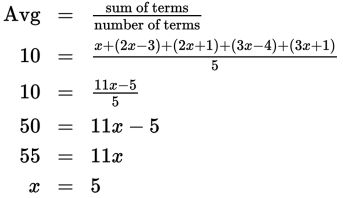# SAT Math Multiple Choice Question 868: Answer and Explanation

### Test Information

Question: 868

13. Five numbers are given by the expressions x, 2x - 3, 2x + 1, 3x - 4, and 3x + 1. If the average (arithmetic mean) of these numbers is 10, what is the mode ofthe numbers?

• A. 10
• B. 11
• C. 12
• D. There is not enough information to determine the value of the mode.

Explanation:

B

Difficulty: Hard

Category: Problem Solving and Data Analysis / Statistics and Probability

Strategic Advice: Try not to overthink a question like this-instead, use what you know about finding an average and see where that takes you.

Getting to the Answer: The average of a set of values is the sum of the values divided by the number of values. Use this definition along with the average given in the question (10) to solve for x:Now, substitute 5 for x in each of the expressions: 5, 2(5) - 3, 2(5) + 1, 3(5) - 4, and 3(5) + 1 give the numbers 5, 7, 11, 11, and 17. The mode of a data set is the number that occurs most often, which in this case is 11. This means (B) is correct.